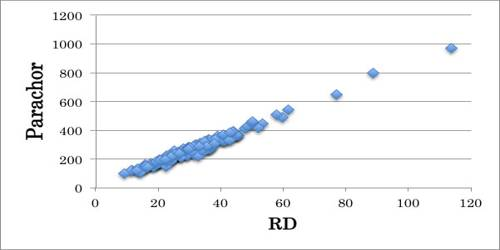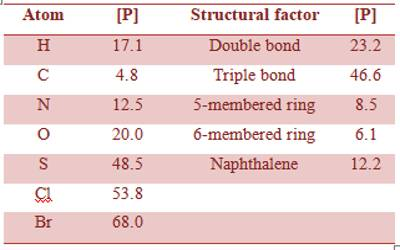# Parachor

Parachor is the molecular weight of liquid times the fourth root of its surface tension, divided by the difference between the density of the liquid and the density of the vapor in equilibrium with it; essentially constant over wide ranges of temperature. An empirical relation between the surface tension of a liquid (γ), its density (D) and that of the vapour (d) was established by Macleod (1923). The relation, known as Macleod equation, is,

γ = C(D – d)4 … … … (1)

where C is a constant, the densities and the surface tensions being measured at the same temperature. The constant C was found to be independent of temperature and the equation was valid over a wide range of temperature. Equation (1) was modified by Sugden (1924) by multiplying both sides of equation (1) by the molecular mass (M) of the liquid.

Mγ = MC (D – d)4 … … … (2)

Equation (2) upon rearrangement gives,

1/4 / (D – d) = MC = [P] … … … (3)

The quantity [P] was found to be constant for a liquid, and Sugden named it as Parachor. If it is assumed that the density of vapor (d) is negligible compared to the density of the liquid at the same temperature, equation (3) may be written as,

1/4 / D = MC = [P]

or, [P] = Vm γ1/4 … … (4)

where vm is the molar volume of the liquid. At the temperature when surface tension is unity, Parachor becomes equal to the molar volume. In other words, the parachor gives a measure of the molar volume at temperatures at which different liquids have the same surface tension.

According to Kopp’s law, the molar volumes are additive, so parachor should also be additive. This was shown to be essentially correct by Sugden. He also showed that parachor is constitutive. From the data for isomeric substances and members of different homologous series Sugden was able to calculate the parachor equivalent for a number of elements and for some structural factors, such as double bond, triple bond, ring structure etc.

The table gives some of the values of parachor equivalent.

Table: Parachor equivalents